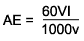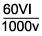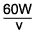# Heat input vs Arc energy in welding

In arc welding processes, heat input (HI) and arc energy (AE) are both measures of how much energy has been supplied to the workpiece to form a weld. They are both measured in units of energy per unit length. In Europe, this tends to be in kJ/mm, whereas in America it tends to be kJ/in.

## Arc energy

Arc energy is the energy supplied by the welding arc to the workpiece before the efficiency of the process is considered. It is calculated by the following equation:where: V is the voltage used, in volts I is the current used, in amperes v is the travel speed of the welding torch, in distance per minute (the units of v dictate whether the arc energy is measured in kJ/mm or kJ/in)

A number of American codes also use the term Heat Input when referencing arc energy.

## Heat input

Heat input (the present best practice term, as it provides a more relevant way of comparing arc welding processes) considers the effect process efficiency has on the energy that actually reaches the workpiece to form the weld. HI is given by the following relationship to AE:

HI = ηAE

where: η is the process efficiency

Each arc welding process has a different efficiency rating. To simplify the rating system, all efficiency factors relate to the efficiency of submerged arc welding.

Process Efficiency
factor
Submerged arc welding (SAW) 1.0
Manual metal arc (MMA)/Shielded metal arc welding (SMAW) 0.8
Cored wire welding/Flux-cored arc welding (FCAW) 0.8
Metal active gas/Metal inert gas (MAG/MIG)/Gas metal arc welding(GMAW) 0.8
Tungsten inert gas (TIG)/Gas tungsten arc welding (GTAW) 0.6
Plasma arc welding 0.6

## Power beam processes

For power beam processes, such as laser or electron beam welding, an arc is not set up between the heat source and the workpiece. The energy transfer (efficiency) factor of the process may vary with the power intensity of the beam, and joint configuration.

In laser welding, the efficiency factor is not included in any terming of ‘heat input’. In electron beam welding, and adjusted current value is entered into the expression for heat input, as an ‘over-current’ is used for full penetration welds to ensure the weld depth. The excess current can be reported by detectors behind the workpiece, so the energy absorbed by the workpiece is calculated when using this adjusted value, rather than the energy supplied by the machine. The effect of heat input on cooling rates for power beam processes is not the same as for arc welding processes, due to the differences in weld shape.

The expression for heat input is similar to that for arc welding processes, and has the same units (kJ/mm or kJ/in):

 Heat Input =, for electron beam welding Heat Input =, for laser welding
 where: V is the voltage used, in kilovolts (kV) I is the adjusted current, in milliamps (mA) W is the laser power, in kilowatts (kW) v is the travel speed, in distance per minute